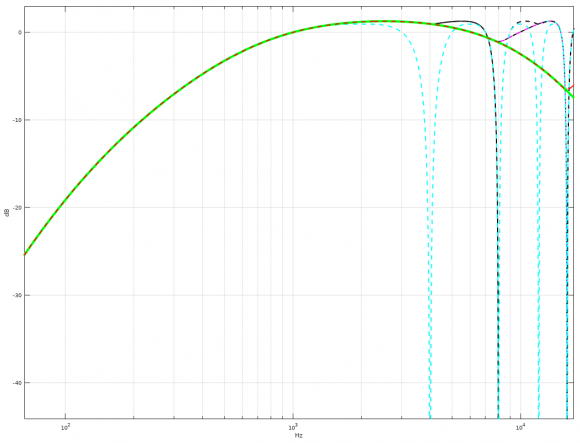## A-Weighting filterStarted by 4 years ago3 replieslatest reply 4 years ago281 views
A-Weighting filter implemented here

uses Bilinear transformation method, which has some issues at high frequency area.

Here's one alternative I made using Octave:

<code>% Octave packages -------------------------------

% other requirements:
% Magnitude Invariance method (MIM) -implementation ( one implementation can be found from https://soar.wichita.edu/handle/10057/1564 )

clf;
format long;

%Sampling Rate
Fs = 44100;

% A-weighting filter frequencies according to IEC/CD 1672.
% Source: <a href="https://www.dsprelated.com/showcode/214.php">https://www.dsprelated.com/showcode/214.php</a>
f1 = 20.598997;
f2 = 107.65265;
f3 = 737.86223;
f4 = 12194.217;

% Analog model -----------------------------------------------------
A1000 = 1.9997;
NUM = [ (2*pi*f4)^2*(10^(A1000/20)) 0 0 0 0];
DEN = conv([1 +4*pi*f4 (2*pi*f4)^2],[1 +4*pi*f1 (2*pi*f1)^2]);
DEN = conv(conv(DEN,[1 2*pi*f3]),[1 2*pi*f2]);

Ds = tf(NUM, DEN);
[Ab, Aa, T] = tfdata(Ds, 'v');

% Digital filter  --------------------------------------------------
%LPF1 (MIM method)
lporder = 1; % 1 = 5th order A-Weighting filter, 2= 6th order ....
w0 = 2 * pi * f4;
bC = 1;
aC = [1 w0];
LP2 = c2dn(AD0^2, 1/Fs, 'mim', lporder, 4096^2, 'lowpass');

% HPF (BLT method)
% HPF1
w0 = 2 * pi * f1;
bC = [w0 0];
aC = [1 w0];

% HPF2
w0 = 2 * pi * f2;
bC = [w0 0];
aC = [1 w0];

% HPF3
w0 = 2 * pi * f3;
bC = [w0 0];
aC = [1 w0];

% Combine filters
FLT = (LP2*HP1*HP2*HP3);

GAINoffset = abs(freqresp(FLT,1000*2*pi));
FLT = FLT/GAINoffset;

% get coefficients
[ad, bd, T] = tfdata(FLT, 'v');
bd

% plot
fs2 = Fs/2;
nf = logspace(0, 5, fs2);

[mag, pha] = bode(Ds,2*pi*nf);
semilogx(nf, 20*log10(abs(mag)), 'color', 'g', 'linewidth', 4.0, 'linestyle', '-');

hold on;

[mag, pha] = bode(FLT,2*pi*nf);
semilogx(nf, 20*log10(abs(mag)), 'color', 'k', 'linewidth', 2.0, 'linestyle', '--');
title('A-Weighting filter');
xlabel('Hz');ylabel('dB');
axis([1 fs2+5000 -200 10]);

legend('Analog model', 'Digital (MIM+BLT)', 'location', 'southeast');
grid on;
</code>

which produces quite accurate magnitude response already for 5th order filter implementation:and works well for low samplerates as well (Fs = 4, 8, 16 and 32 kHz):[ - ]I gave a paper that addressed this problem at the 2010 comp.dsp conference.

I have it in pdf format, but I am not sure how to submit it.

Here are some of the ideas.

If you split the high pass portion of the A weight filter from the low pass part, then you can use BZT for the high pass section and get a very good fit for this part. This leaves you with the double real poles at 12.2K. This portion is fitted with a magnitude squared approach.

Therefore rewrite

HA(s) = H123(s) H4(s)

HA(s) = [s4/[(s+w1)2(s+w2)(s+w3)] *

w42 /(s+w4)2

H123(s) -> HBZT123(z) Use BZT

H4(s) = [w4 /(s+w4)] * [w4 /(s+w4)]

Use two second order HFIR (z) where

1st section uses w0 = 0, w1 = wp, w2 = p-wp

2nd section uses w0 = 0, w1 = p/3, w2 = 2p/3

* Using two different matching criteria spreads the error

* w0, w1, w2 are not the A weight definitions

* wp = 76618.5439, the pole location Magnitude squared

If HFIR(z) = b0 + b1z-1 + b2z-2 then

|HFIR(z)|2= b02 + b12 + b22 + 2b0b1cos w +

2b0b2cos 2w + 2b1b2cos w

We now compute the filter by matching three points. This is difficult to factor for arbitrary w, but the above choices are easier to solve.

Part of this idea comes from this paper:

David Gunness & Ojas Chauhan, Optimizing the magnitude response of matched Z transform filters (MZTi) for Loudspeaker Equalization, Proceedings AES 32nd International Conference 21-23 Sept 2007

Al Clark

[ - ]